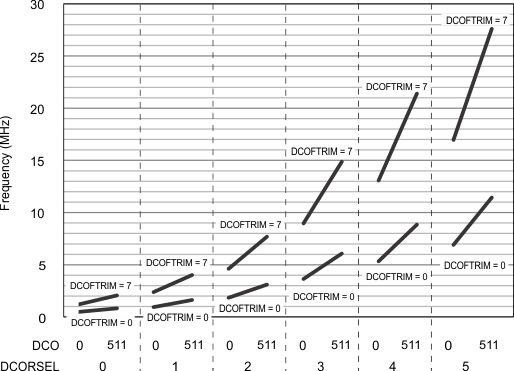SLAS942E November   2015  – December 2019

PRODUCTION DATA.

1. 1Device Overview
2. 2Revision History
3. 3Device Comparison
4. 4Terminal Configuration and Functions
5. 5Specifications
1. 5.1       Absolute Maximum Ratings
2. 5.2       ESD Ratings
3. 5.3       Recommended Operating Conditions
4. 5.4       Active Mode Supply Current Into VCC Excluding External Current
5. 5.5       Active Mode Supply Current Per MHz
6. 5.6       Low-Power Mode LPM0 Supply Currents Into VCC Excluding External Current
7. 5.7       Low-Power Mode (LPM3 and LPM4) Supply Currents (Into VCC) Excluding External Current
8. 5.8       Low-Power Mode LPMx.5 Supply Currents (Into VCC) Excluding External Current
9. 5.9       Typical Characteristics - Low-Power Mode Supply Currents
10. Table 5-1 Typical Characteristics – Current Consumption Per Module
11. 5.10      Thermal Resistance Characteristics
12. 5.11      Timing and Switching Characteristics
1. 5.11.1  Power Supply Sequencing
2. 5.11.2  Reset Timing
3. 5.11.3  Clock Specifications
4. 5.11.4  Digital I/Os
5. 5.11.5  VREF+ Built-in Reference
6. 5.11.6  Timer_A
7. 5.11.7  eUSCI
9. 5.11.9  CapTIvate
10. 5.11.10 FRAM
11. 5.11.11 Debug and Emulation
6. 6Detailed Description
7. 7Applications, Implementation, and Layout
1. 7.1 Device Connection and Layout Fundamentals
2. 7.2.2 CapTIvate Peripheral
1. 7.2.2.1 Device Connection and Layout Fundamentals
2. 7.2.2.2 Measurements
3. 7.3 CapTIvate Technology Evaluation
8. 8Device and Documentation Support
9. 9Mechanical, Packaging, and Orderable Information

• DA|32
• RHB|32
• RHB|32

Table 5-6 DCO Frequency

over recommended operating free-air temperature (unless otherwise noted) (also see Figure 5-5)
PARAMETER TEST CONDITIONS VCC TYP UNIT
fDCO, 16MHz DCO frequency, 16 MHz DCORSEL = 101b, DISMOD = 1b, DCOFTRIMEN = 1b, DCOFTRIM = 000b, DCO = 0 3 V 7.46 MHz
DCORSEL = 101b, DISMOD = 1b, DCOFTRIMEN = 1b, DCOFTRIM = 000b, DCO = 511 12.26
DCORSEL = 101b, DISMOD = 1b, DCOFTRIMEN = 1b, DCOFTRIM = 111b, DCO = 0 17.93
DCORSEL = 101b, DISMOD = 1b, DCOFTRIMEN = 1b, DCOFTRIM = 111b, DCO = 511 29.1
fDCO, 12MHz DCO frequency, 12 MHz DCORSEL = 100b, DISMOD = 1b, DCOFTRIMEN = 1b, DCOFTRIM = 000b, DCO = 0 3 V 5.75 MHz
DCORSEL = 100b, DISMOD = 1b, DCOFTRIMEN = 1b, DCOFTRIM = 000b, DCO = 511 9.5
DCORSEL = 100b, DISMOD = 1b, DCOFTRIMEN = 1b, DCOFTRIM = 111b, DCO = 0 13.85
DCORSEL = 100b, DISMOD = 1b, DCOFTRIMEN = 1b, DCOFTRIM = 111b, DCO = 511 22.5
fDCO, 8MHz DCO frequency, 8 MHz DCORSEL = 011b, DISMOD = 1b, DCOFTRIMEN = 1b, DCOFTRIM = 000b, DCO = 0 3 V 3.91 MHz
DCORSEL = 011b, DISMOD = 1b, DCOFTRIMEN = 1b, DCOFTRIM = 000b, DCO = 511 6.49
DCORSEL = 011b, DISMOD = 1b, DCOFTRIMEN = 1b, DCOFTRIM = 111b, DCO = 0 9.5
DCORSEL = 011b, DISMOD = 1b, DCOFTRIMEN = 1b, DCOFTRIM = 111b, DCO = 511 15.6
fDCO, 4MHz DCO frequency, 4 MHz DCORSEL = 010b, DISMOD = 1b, DCOFTRIMEN = 1b, DCOFTRIM = 000b, DCO = 0 3 V 2.026 MHz
DCORSEL = 010b, DISMOD = 1b, DCOFTRIMEN = 1b, DCOFTRIM = 000b, DCO = 511 3.407
DCORSEL = 010b, DISMOD = 1b, DCOFTRIMEN = 1b, DCOFTRIM = 111b, DCO = 0 4.95
DCORSEL = 010b, DISMOD = 1b, DCOFTRIMEN = 1b, DCOFTRIM = 111b, DCO = 511 8.26
fDCO, 2MHz DCO frequency, 2 MHz DCORSEL = 001b, DISMOD = 1b, DCOFTRIMEN = 1b, DCOFTRIM = 000b, DCO = 0 3 V 1.0225 MHz
DCORSEL = 001b, DISMOD = 1b, DCOFTRIMEN = 1b, DCOFTRIM = 000b, DCO = 511 1.729
DCORSEL = 001b, DISMOD = 1b, DCOFTRIMEN = 1b, DCOFTRIM = 111b, DCO = 0 2.525
DCORSEL = 001b, DISMOD = 1b, DCOFTRIMEN = 1b, DCOFTRIM = 111b, DCO = 511 4.25
fDCO, 1MHz DCO frequency, 1 MHz DCORSEL = 000b, DISMOD = 1b, DCOFTRIMEN = 1b, DCOFTRIM = 000b, DCO = 0 3 V 0.5319 MHz
DCORSEL = 000b, DISMOD = 1b, DCOFTRIMEN = 1b, DCOFTRIM = 000b, DCO = 511 0.9029
DCORSEL = 000b, DISMOD = 1b, DCOFTRIMEN = 1b, DCOFTRIM = 111b, DCO = 0 1.307
DCORSEL = 000b, DISMOD = 1b, DCOFTRIMEN = 1b, DCOFTRIM = 111b, DCO = 511 2.21VCC = 3 V TA = –40°C to 85°C
Figure 5-5 Typical DCO Frequency

Table 5-7 lists the characteristics of the REFO.